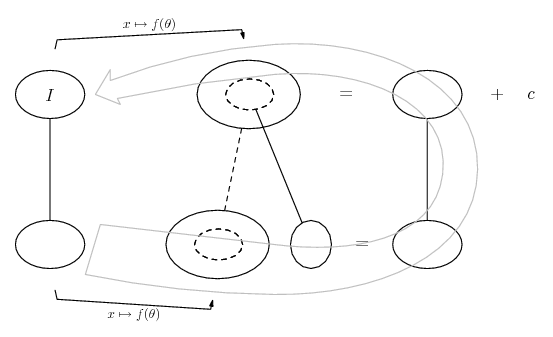# Thread: Integration Steps for integral of 1/((x^2 + a^2)*Sqrt[x^2 + a^2 + b^2]) wrt x?

1. ## Integration Steps for integral of 1/((x^2 + a^2)*Sqrt[x^2 + a^2 + b^2]) wrt x?

Hi

I want to understand the steps required to compute the indefinite integral of 1/((x^2 + a^2)*Sqrt[x^2 + a^2 + b^2]).

Wolfram Mathematica gives the solution to be ArcTan[(b*x)/(a*Sqrt[a^2 + b^2 + x^2])]/(a*b)

I have tried various substitutions but cannot get anywhere near this. I can easily derive the integral of 1/(x^2+a^2) to get arctan - so Im quite proficient in doing such integrals - but I cannot find a good substitution for this one.

Any help would be greatly appreciated
Best
Lisse

2. ## Re: Integration Steps for integral of 1/((x^2 + a^2)*Sqrt[x^2 + a^2 + b^2]) wrt x?

Yes. You do know about the 'show steps' button on Wolfram, I suppose? But it doesn't seem to be coming up with the goods, that's true: integrate 1/((x^2 + a^2)*Sqrt[x^2 + a^2 + b^2]) - Wolfram|Alpha.

Well, you can always differentiate the integral! The inside function of the main chain rule process is clearly enough,

$\displaystyle \frac{b}{a} \frac{x}{\sqrt{x^2 + a^2 + b^2}}$

... so we could fantasise about having had the inspiration to manipulate the integrand into a product of that and its derivative...

$\displaystyle \frac{1}{(x^2 + a^2) \sqrt{x^2 + a^2 + b^2}}$

$\displaystyle =\ \frac{1}{(x^2 + a^2)}\ \frac{x^2 + a^2 + b^2}{(x^2 + a^2 + b^2)^{3/2}}$

$\displaystyle =\ \frac{a^2 + b^2}{a^2(x^2 + a^2 + b^2) + b^2 x^2}\ \frac{x^2 + a^2 + b^2}{(x^2 + a^2 + b^2)^{3/2}}$

$\displaystyle =\ \frac{x^2 + a^2 + b^2}{a^2(x^2 + a^2 + b^2) + b^2 x^2}\ \frac{a^2 + b^2}{(x^2 + a^2 + b^2)^{3/2}}$

$\displaystyle =\ \frac{a}{b}\ \frac{x^2 + a^2 + b^2}{a^2(x^2 + a^2 + b^2) + b^2 x^2}\ \frac{b}{a}\ \frac{a^2 + b^2}{(x^2 + a^2 + b^2)^{3/2}}$

$\displaystyle =\ \frac{1}{ab}\ \frac{x^2 + a^2 + b^2}{(x^2 + a^2 + b^2) + \frac{b^2}{a^2} x^2}\ \frac{b}{a}\ \frac{a^2 + b^2}{(x^2 + a^2 + b^2)^{3/2}}$

$\displaystyle =\ \frac{1}{ab}\ \frac{1}{1 + \frac{b^2}{a^2} \frac{x^2}{x^2 + a^2 + b^2}}\ \frac{b}{a}\ \frac{a^2 + b^2}{(x^2 + a^2 + b^2)^{3/2}}$

$\displaystyle =\ \displaystyle{\frac{1}{ab}\ \frac{1}{1 + [\frac{b}{a} \frac{x}{\sqrt{x^2 + a^2 + b^2}}]^2}\ \frac{b}{a}\ \frac{a^2 + b^2}{(x^2 + a^2 + b^2)^{3/2}}}$

I don't quite see how lines 2 and 3 equate, but they seem to.

Just in case a picture helps..... where (key in spoiler) ...

Spoiler:... is the chain rule. Straight continuous lines differentiate downwards (integrate up) with respect to the main variable (in this case x or theta), and the straight dashed line similarly but with respect to the dashed balloon expression (the inner function of the composite which is subject to the chain rule).

The general drift is...Full size

__________________________________________________ _______________________

Don't integrate - balloontegrate!

Balloon Calculus; standard integrals, derivatives and methods

Balloon Calculus Drawing with LaTeX and Asymptote!

#### Search Tags

1 or x2, a2sqrtx2, integral, integration, steps, wrt